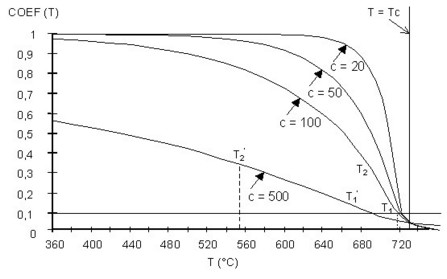# Temperature coefficient

## Introduction

The models provided for B(H) curves, depending on the temperature, with exponential decay, are defined using:

• the previous models provided for soft materials
• a COEF(T) temperature coefficient, built on the basis of two exponential functions (detailed description is in following blocks)

## Temperature coefficient

The COEF(T) temperature coefficient is defined by two exponentials:

• the first, with a negative curvature, is used when this coefficient ranges from 1 to 0.1 (T < T1)
• the second, with a positive curvature, is utilized in the neighborhood of the Curie point, when this coefficient ranges from 0.1 to 0 (T < T1 )

The shape of the COEF(T) temperature coefficient is represented in the figure below.## Mathematical model

The COEF(T) temperature coefficient is defined in the following way:

for T < T1: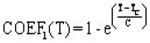where for the temperature T1 we have COEF1(T1) = 0.1

The last relationship can be equally written as: T1 -Tc =C ln 0.9

for T > T1: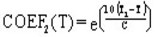where the quantity T2 has the value so that the connection of the two exponentials could be effected in T1, i.e. COEF2(T1) = 0.1

This last condition can be equally written as: T2 -T1 =0.1C ln 0.1

where:

• Tc is the Curie temperature °C
• C is the temperature constant °C

## Exact mathematic expression

The expression of COEF(T) used above between 1 and 0,1 is approximate. The accurate expression :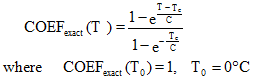As Tc is elevated, around 1000 K, we use the approximation: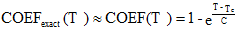And at Td0 = 0°C: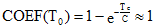## Example

The shape of the COEF(T) function is represented in the figure below.

The decrease of the COEF(T) coefficient is more or less rapid following the value of the C temperature constant supplied by the user.